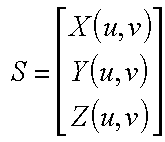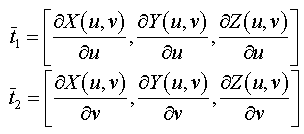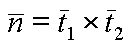# Normals of non planar Surfaces

## Example : parametric surface

Not all surfaces are given as planar facets. A common example of such a surface is called a parametric surface. For a parametric surface the three-space coordinates are determined by functions of two parameters, u and v in our case. For parametric surfaces two vectors in the tangent plane can be found by computing partial derivatives as follows. And the normal is computed as before:Web www.vrarchitect.net